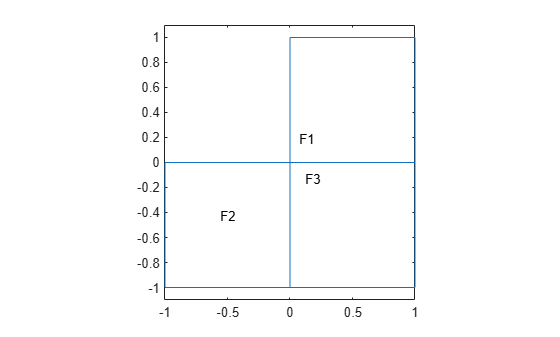# findThermalProperties

Find thermal material properties assigned to a geometric region

## Syntax

``tmpa = findThermalProperties(thermalmodel.MaterialProperties,RegionType,RegionID)``

## Description

example

````tmpa = findThermalProperties(thermalmodel.MaterialProperties,RegionType,RegionID)` returns thermal material properties `tmpa` assigned to the specified region.```

## Examples

collapse all

Create a transient thermal model that has three faces.

```thermalmodel = createpde("thermal","transient"); geometryFromEdges(thermalmodel,@lshapeg); pdegplot(thermalmodel,"FaceLabels","on") ylim([-1.1,1.1]) axis equal```For face 1, specify the following thermal properties:

• Thermal conductivity is $10\text{\hspace{0.17em}}\mathrm{W}/\left(\mathrm{m}{\cdot }^{\circ }\mathit{C}\right)$

• Mass density is $1\text{\hspace{0.17em}}\mathrm{kg}/{\mathrm{m}}^{3}$

• Specific heat is $0.1\text{\hspace{0.17em}}\mathrm{J}/\left(\mathrm{kg}{\cdot }^{\circ }\mathrm{C}\right)$

```thermalProperties(thermalmodel,"ThermalConductivity",10,... "MassDensity",1,... "SpecificHeat",0.1,... "Face",1);```

For face 2, specify the following thermal properties:

• Thermal conductivity is $20\text{\hspace{0.17em}}\mathrm{W}/\left(\mathrm{m}{\cdot }^{\circ }\mathit{C}\right)$

• Mass density is $2\text{\hspace{0.17em}}\mathrm{kg}/{\mathrm{m}}^{3}$

• Specific heat is $0.2\text{\hspace{0.17em}}\mathrm{J}/\left(\mathrm{kg}{\cdot }^{\circ }\mathrm{C}\right)$

```thermalProperties(thermalmodel,"ThermalConductivity",20,... "MassDensity",2,... "SpecificHeat",0.2,... "Face",2);```

For face 3, specify the following thermal properties:

• Thermal conductivity is $30\text{\hspace{0.17em}}\mathrm{W}/\left(\mathrm{m}{\cdot }^{\circ }\mathit{C}\right)$)

• Mass density is $3\text{\hspace{0.17em}}\mathrm{kg}/{\mathrm{m}}^{3}$

• Specific heat is $0.3\text{\hspace{0.17em}}\mathrm{J}/\left(\mathrm{kg}{\cdot }^{\circ }\mathrm{C}\right)$

```thermalProperties(thermalmodel,"ThermalConductivity",30,... "MassDensity",3,... "SpecificHeat",0.3,... "Face",3);```

Check the material properties specification for face 1.

```mpaFace1 = findThermalProperties(thermalmodel.MaterialProperties, ... "Face",1)```
```mpaFace1 = ThermalMaterialAssignment with properties: RegionType: 'face' RegionID: 1 ThermalConductivity: 10 MassDensity: 1 SpecificHeat: 0.1000 ```

Check the heat source specification for faces 2 and 3.

```mpa = findThermalProperties(thermalmodel.MaterialProperties, ... "Face",[2,3]); mpaFace2 = mpa(1)```
```mpaFace2 = ThermalMaterialAssignment with properties: RegionType: 'face' RegionID: 2 ThermalConductivity: 20 MassDensity: 2 SpecificHeat: 0.2000 ```
`mpaFace3 = mpa(2)`
```mpaFace3 = ThermalMaterialAssignment with properties: RegionType: 'face' RegionID: 3 ThermalConductivity: 30 MassDensity: 3 SpecificHeat: 0.3000 ```

Create a geometry that consists of three stacked cylinders and include the geometry in a thermal model.

`gm = multicylinder(10,[1 2 3],"ZOffset",[0 1 3])`
```gm = DiscreteGeometry with properties: NumCells: 3 NumFaces: 7 NumEdges: 4 NumVertices: 4 Vertices: [4x3 double] ```
```thermalmodel = createpde("thermal"); thermalmodel.Geometry = gm; pdegplot(thermalmodel,"CellLabels","on","FaceAlpha",0.5)```Thermal conductivity of the cylinder `C1` is $10\text{\hspace{0.17em}}\mathrm{W}/\left(\mathrm{m}{\cdot }^{\circ }\mathrm{C}\right)$.

`thermalProperties(thermalmodel,"ThermalConductivity",10,"Cell",1);`

Thermal conductivity of the cylinder `C2` is $20\text{\hspace{0.17em}}\mathrm{W}/\left(\mathrm{m}{\cdot }^{\circ }\mathrm{C}\right)$.

`thermalProperties(thermalmodel,"ThermalConductivity",20,"Cell",2);`

Thermal conductivity of the cylinder `C3` is $30\text{\hspace{0.17em}}\mathrm{W}/\left(\mathrm{m}{\cdot }^{\circ }\mathrm{C}\right)$.

`thermalProperties(thermalmodel,"ThermalConductivity",30,"Cell",3);`

Check the material properties specification for cell 1:

```mpaCell1 = findThermalProperties(thermalmodel.MaterialProperties, ... "Cell",1)```
```mpaCell1 = ThermalMaterialAssignment with properties: RegionType: 'cell' RegionID: 1 ThermalConductivity: 10 MassDensity: [] SpecificHeat: [] ```

Check the heat source specification for cells 2 and 3:

```mpa = findThermalProperties(thermalmodel.MaterialProperties,"Cell",2:3); mpaCell2 = mpa(1)```
```mpaCell2 = ThermalMaterialAssignment with properties: RegionType: 'cell' RegionID: 2 ThermalConductivity: 20 MassDensity: [] SpecificHeat: [] ```
`mpaCell3 = mpa(2)`
```mpaCell3 = ThermalMaterialAssignment with properties: RegionType: 'cell' RegionID: 3 ThermalConductivity: 30 MassDensity: [] SpecificHeat: [] ```

## Input Arguments

collapse all

Material properties of the model, specified as the `MaterialProperties` property of a thermal model.

Example: `thermalmodel.MaterialProperties`

Geometric region type, specified as `"Face"` or `"Cell"`.

Example: `findThermalProperties(thermalmodel.MaterialProperties,"Cell",1)`

Data Types: `char` | `string`

Geometric region ID, specified as a vector of positive integers. Find the region IDs by using `pdegplot`.

Example: `findThermalProperties(thermalmodel.MaterialProperties,"Face",1:3)`

Data Types: `double`

## Output Arguments

collapse all

Material properties assignment, returned as a `ThermalMaterialAssignment` object. See ThermalMaterialAssignment Properties.

## Version History

Introduced in R2017a